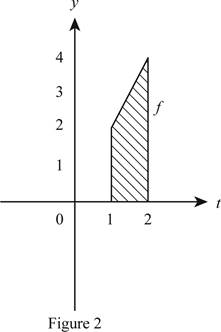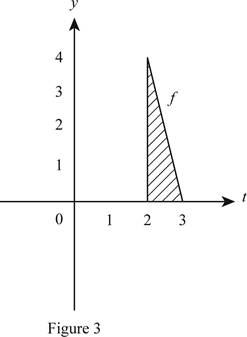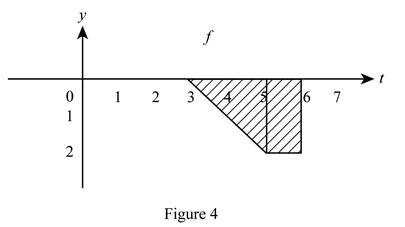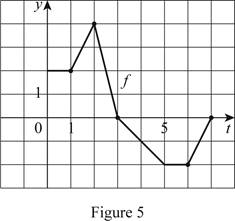# the value of g ( 0 ) , g ( 1 ) , g ( 2 ) , g ( 3 ) ,and g ( 6 ) .### Single Variable Calculus: Concepts...

4th Edition
James Stewart
Publisher: Cengage Learning
ISBN: 9781337687805### Single Variable Calculus: Concepts...

4th Edition
James Stewart
Publisher: Cengage Learning
ISBN: 9781337687805

#### Solutions

Chapter 5.4, Problem 3E

(a)

To determine

## To determine the value of g(0), g(1), g(2), g(3),and g(6).

Expert Solution

The value of g(0) is 0.

The value of g(1) is 2_.

The value of g(2) is 5_.

The value of g(3) is 7_.

The value of g(6) is 3_.

### Explanation of Solution

Given information:

The integral function is g(x)=0xf(t)dt.

Calculation:

Show the integral function as below.

g(x)=0xf(t)dt (1)

Here, g(x) is area under the graph of f from a to x and f(t) is function of t.

Substitute 0 for x in Equation (1).

g(0)=00f(t)dt=0

Therefore, the g(0) is 0_.

Draw the graph for calculation of g(1) as in Figure 1.Determine g(1) using Equation (1).

Substitute 1 for x in Equation (1).

g(1)=01f(t)dt (2)

Refer to Figure (1).

Modify Equation (2).

g(1)=bh

Substitute 1 for b and 2 for h.

g(1)=bh=(1)(2)=2

Therefore, the g(1) is 2_.

Draw the graph for calculation of g(2) as in Figure 2.Determine g(2) using Equation (1).

Substitute 2 for x in Equation (1).

g(2)=02f(t)dt=01f(t)dt+12f(t)dt=g(1)+12f(t)dt (3)

Refer to figure 2.

The area of shaded triangle and rectangle is the function of t with limits 1 to 2.

Substitute 2 for g(1) and (12bh) for 12f(t)dt in Equation (3).

g(2)=g(1)+12f(t)dt=2+(12bh)

Substitute 1 for b and 2 for h.

g(2)=2+(1)(2)+12(1)(2)=2+2+1=5

Therefore, the g(2) is 5_.

Draw the graph for calculation of g(3) as in Figure 3.Determine g(3) using Equation (1).

Substitute 3 for x in Equation (1).

g(3)=03f(t)dt=01f(t)dt+12f(t)dt+23f(t)dt=g(2)+23f(t)dt (4)

Refer to Figure 3.

The area of shaded triangle is the function of t with limits 2 to 3.

Substitute 5 for g(2) and (12bh) for 32f(t)dt in Equation (4).

g(3)=g(2)+32f(t)dt=5+12(b)(h)=12bh

Substitute 1 for b and 4 for h.

g(3)=12bh=5+12(1)(4)=7

Therefore, the g(3) is 7_.

Draw the graph for calculation of g(6) as in Figure 4.Determine g(6) using Equation (1).

g(6)=36f(t)dt=g(3)+34f(t)dt+45f(t)dt+56f(t)dt=g(5)+56f(t)dt (5)

Refer to Figure 4.

Area of shaded portion is the function of t with limits 5 to 6.

g(6)=g(3)+56f(t)dt=7+((12)bh+b1h1)

Substitute 2for b, 2 for h, 1 for b1, and 2 for h1.

g(6)=7+(12(2)(2)+(1)(2))=74=3

Therefore, the g(6) is 3_.

(b)

To determine

### The interval g.

Expert Solution

The function g is increasing at the interval (0,3)_.

### Explanation of Solution

Given information:

The integral function is g(x)=0xf(t)dt.

Calculation:

Refer Part (a).

The value of g is increasing from the interval 0 to 3.

Therefore, the function g is increasing at the interval (0,3)_.

(c)

To determine

### The maximum value of g.

Expert Solution

The maximum value of g is lies at x=3_.

### Explanation of Solution

Given information:

The integral function is g(x)=0xf(t)dt.

Calculation:

Refer part (a) calculation

Maximum value of g lies at x=3

Therefore, the maximum value of g lies at x=3_.

(d)

To determine

Expert Solution

### Explanation of Solution

The graph for function f is shown in Figure 1.

Plot the graph for function f using the calculated values of 0, 2,5,7,3 for the functions g(0), g(1), g(2), g(3), and g(6) respectively.

Draw the graph for function of f as in Figure (5).### Have a homework question?

Subscribe to bartleby learn! Ask subject matter experts 30 homework questions each month. Plus, you’ll have access to millions of step-by-step textbook answers!